Study Guide

# Points, Vectors, and Functions - Vectors: A New Kind of Animal

## Vectors: A New Kind of Animal

Imagine we're walking down the street after a tasty supper. We look to our left, and we sight a minotaur ambling down the street with a cup of joe in hand. Distracted, we trip over an overgrown root breaking through the sidewalk. Clumsy us. We land on our backs, only to see, of all things, a griffin flying high overhead. We squint, wondering if our lunch was poisoned, and try to make sense of what we've seen.Of course, like any studious scholars, we run home and look up what we saw on Shmoop, and realize that the Minotaur is a harmless half man, half bull, while the griffin is an eagle/lion hybrid out for a post-evening joy flight. It clicks: we saw single, harmless creatures each composed of two animals.

Turns out this is a good way to think about vectors. They are mathematical objects composed of two, easily recognizable parts, magnitude and direction.

### Sample Problem

Velocity is a vector, since it has magnitude, or the speed, and direction. "60 miles per hour due east" is a velocity.

### Sample Problem

Speed is not a vector. It has magnitude but doesn't include a direction. "60 miles per hour" is a speed, not a velocity.

Most of the vectors that show up in calculus are two-dimensional (2-D). Much like these mythologically astounding sightings, a 2-D vector is like a mutant point. We write a point on the x,y-plane as an ordered pair of coordinates (x, y).
We write a 2-D vector as an ordered pair of components

(notice the pointy brackets instead of parentheses).

We can visualize a 2-D vector as a line segment with an arrow on one end:The end of the line segment with the arrow is called the head of the vector. The other end is called the tail.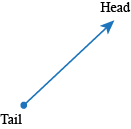The head end shows which way the vector is going. Like animals and arrows, vectors travel head first.The components x and y of the vector are like the Δ x and Δ y we use when discussing slope. To get from the tail to the head of the vector , we go over by x and up by y: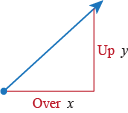When visualizing a 2-D vector as a line segment with its tail at the origin,

• the magnitude of the vector is the length of the line segment:
•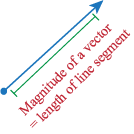• the direction of the vector is the counterclockwise angle between the positive x-axis and the line segment:
•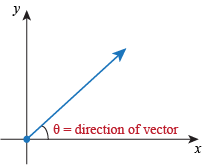We'll go into more depth about magnitude and direction shortly.

For any point P in the xy-plane, we find a corresponding vector by drawing an arrow with its tail at the origin and its head at P.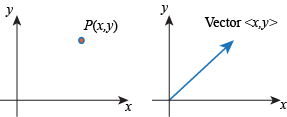For any 2-D vector whose tail is at the origin, we find a corresponding point by drawing a dot at the head of the vector: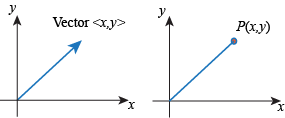We can also say, "there's a one-to-one correspondence between 2-D vectors and points in the xy-plane."

Unless there's a good reason not to, we usually assume a vector has its tail at the origin.

• ### Magnitude

With an understanding that vectors are simply mathematical equivalents of mythological hybrid beasts, we'll investigate their parts to see if we can understand them better. Surely, with the help of the minotaur and griffin vectors, the calculus bear stands no chance against us, right?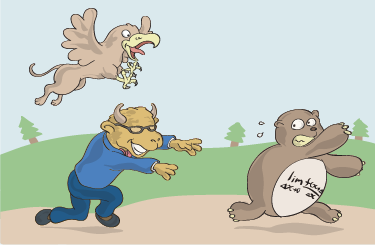The magnitude of a vector is like the body of the vector. We know how much vector is there. We use the notation
||(insert vector here)|| for the magnitude of the vector . This is like the notation for absolute value, but we use two little lines on each side instead of one line.

When we draw a vector as an arrow in the xy-plane, the length of the arrow is the magnitude of the vector.

This length also happens to be the hypotenuse of a right triangle whose legs have lengths x and y. We can find the magnitude of the vector using the Pythagorean Theorem.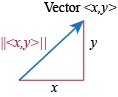The magnitude is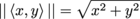.

Be Careful: When finding the magnitude of a vector with negative components, either

• use parentheses and square the components correctly, or

• drop the negative signs altogether.

Since finding magnitude requires squaring the components anyway, it doesn't matter if the components are negative or positive.

Magnitude is a lot like absolute value in this way.

Whether a number is positive or negative doesn't affect its absolute value: |-5| = |5|.

Similarly, whether the components of a vector are positive or negative doesn't affect its magnitude: ||<1, 2>|| = ||<-1, 2>|| = ||<1, -2>|| = ||<-1, -2>||.

Look at the vectors on a graph. The arrows are all the same length; they're pointing different directions:

If a vector lies flat along the x- or y-axis, we don't need to use the Pythagorean Theorem to find its magnitude. Such a vector will have only one component that isn't zero, and that component will be the magnitude of the vector.

• ### Direction

Since we know all about the bodies, err, magnitudes of vectors, we now need to look at the directions of vectors. We can equate the direction of the vector to the head of the griffin or the minotaur. This is especially convenient because the head of the vector tells its direction.

For vectors with tails on the origin, we usually measure the direction of a vector as the counterclockwise angle from the positive x-axis to the vector.

We would say this vector has direction θ:When finding the direction of a vector, use radians unless told otherwise. We recommend reviewing the unit circle before continuing.

When given a vector and asked to find its direction, the first thing we figure out is which quadrant the vector is in. Then we check to see if our answer makes sense.

When a vector is in Quadrant I, we can find its direction θ in one step: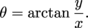When a vector isn't in Quadrant I, we can't trust the arctan function to give the correct direction of the vector. Remember that arctan has a range (-π/2, π/2). Instead, we use the arctan function to find the reference angle of the vector. If we know the reference angle and which quadrant the vector is in, we can find the direction of the vector.

The reference angle is the smallest angle between the vector and the x-axis.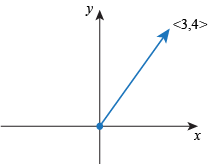We can think of the reference angle as the "family" the angle is in, whether it's one of the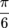angles, one of the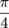angles, and so on.

The reference angle is always between 0 and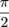radians.

When a vector lies along the x- or y-axis, we don't have to bother with the arctan stuff. All we have to do is see whether the vector is at an angle of 0,, π, or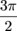;

• ### Scaling Vectors

What's one apple plus another apple? Two apples.

What's two oranges less one orange? One orange.

What's one griffin plus one minotaur? Any mythology buff could tell you it's a griffotaur, of course.

Adding and subtracting vectors is something like adding the griffin and the minotaur. It's possible to add two vectors together or subtract one vector from another. We get another vector in its place. To add vectors, we add the x-components to each other and add the y-components to each other.

### Sample Problem

To add the vectors <3, 4> and <5, 6> we add the first components together and add the second components together:

< 3, 4> + <5, 6 > = <8, 10>.

### Sample Problem

To subtract, we subtract the x-components and then subtract the y-components.

<3, 4> – <5, 6> = <-2, -2>.

When we add or subtract vectors, remember to deal with the x-components and the y-components separately. Why? Going back to counting fruit, apples are the x-component and oranges are the y-component of vectors. We can't add apples to oranges, but we can add apples to apples and oranges to oranges.

Now we're going to work with multiplication of a vector by a real number. To multiply a vector by a number, we multiply each component of the vector by that number.

Multiply the minotaur vector by 2. What do we get? A minotaur twice the size. Likewise, if we multiply the griffin by 0.01, we get a pint-sized, cute griffin to take home with us.

When we multiply a vector by a real number, we call the number a scalar.

We call the real number a scalar because it scales the vector to be a different size. If the scalar is negative it will also change the direction of the vector.

### Sample Problem

Take the vector <1, 2>. This vector has magnitude √5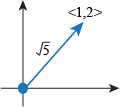Now multiply the vector by 3 to get a new vector: <1, 2> → <3, 6>. The magnitude of the new vector is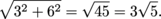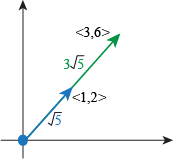The original vector <1, 2> had magnitude √5, The magnitude of the new vector <3, 6> is 3 times greater than the magnitude of the original vector, <1, 2>. In multiplying the vector by 3, we scaled the arrow to be 3 times longer.

• ### Unit Vectors

We've been through apples, oranges and mythological creatures unlike anyone has ever seen. But we still don't have a quick way to compare the magnitude of a vector before and after we scale it.

To do this, we have to resort back to one of the oldest tricks in the great book of mathematics: the number 1.

What's the vector equivalent of the number 1? It's a unit vector, which is a vector that has magnitude equal to 1.

Turns out that we can scale any vector to get a unit vector pointing in the same direction. If the vector
has magnitude less than 1, we stretch it.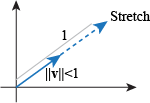If the vector has magnitude greater than 1, we shrink it.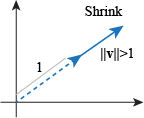The process of scaling a vector to get a unit vector pointing in the same direction is called normalization.

• ### Vector Notation

When Juliet said "that which we call a rose / by any other name would smell as sweet" she could have been talking about math.
As Romeo would retain his "dear perfection" if he were called something else, a vector retains its x and y components regardless of how we write the vector.

Vectors are much less complicated than people. We've never heard of anyone dying for vector love.

The purpose of vector notation is to keep the x and y components straight. Here are some of the many ways we can write a vector whose x-component is 5 and whose y-component is 4.

• We can use pointy brackets. The x-component comes first and the y-component second:
<5, 4>
• We can use bold letters to denote the components. The coefficient in front of the letter i is the x-component and the coefficient in front of the letter j is the y-component:
5i + 4j
• We can use a matrix where the upper entry is the x-component and the lower entry is the y-component:
( 5
4  )
• We can use parentheses instead of pointy brackets, although this notation can lead to confusion between vectors and points:
(5, 4)
• When we see this notation, we need to be careful to discern the difference between a vector and a point. Remember a 2-D point is an ordered pair of coordinates. A 2-D vector is represented by two coordinates that denote the vectors magnitude and direction.
• ### (More than 2)-Dimensional Vectors

We have one more detail to discuss about vectors: dimensionality.

Vectors can be more than 2-dimensional. A 3-dimensional vector has x-, y-, and z-components:

(x, y, z).

More generally, an n-dimensional vector has n different components:

(x1, x2, ..., xn).

Finding the direction of such a vector is a little more complicated than in the 2-D case. However, finding the magnitude isn't any more complicated: we square all the components and add them up. The magnitude of the n-dimensional vector (x1, x2, ..., xn) is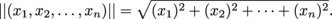Vectors can have any positive integer number of dimensions. Though the notation can get hairy when using letter-symbols for denoting vectors with more than 26 dimensions, those vectors still exist. They are used all the time in complex problems solved by computer algorithms. It's also possible to have infinite-dimensional vectors.